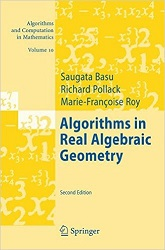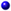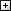Processing ......FreeComputerBooks.com Free Computer, Mathematics, Technical Books and Lecture Notes, etc.

Algorithms in Real Algebraic Geometry
Want to know Runways information of a particular airport? Click here to find out.
• Title Algorithms in Real Algebraic Geometry
• Author(s) Saugata Basu (Author), Richard Pollack (Author), Marie-Françoise Roy (Author)
• Publisher: Springer; 2nd edition (August 21, 2006)
• Hardcover 662 pages
• eBook PDF (672 pages)
• Language: English
• ISBN-10: 3540330984
• ISBN-13: 978-3540330981Book Description

This is the first graduate textbook on the algorithmic aspects of real algebraic geometry. The main ideas and techniques presented form a coherent and rich body of knowledge. Mathematicians will find relevant information about the algorithmic aspects. Researchers in computer science and engineering will find the required mathematical background. Being self-contained the book is accessible to graduate students and even, for invaluable parts of it, to undergraduate students. This second edition contains several recent results on discriminants of symmetric matrices and other relevant topics.

The monograph gives a self-contained detailed exposition of the algorithmic real algebraic geometry. In general, the monograph is well written and will be useful both for beginners and for advanced readers, who work in real algebraic geometry or apply its methods in other fields.

The algorithmic problems of real algebraic geometry such as real root counting, deciding the existence of solutions of systems of polynomial equations and inequalities, finding global maxima or deciding whether two points belong in the same connected component of a semi-algebraic set appear frequently in many areas of science and engineering. In this first-ever graduate textbook on the algorithmic aspects of real algebraic geometry, the main ideas and techniques presented form a coherent and rich body of knowledge, linked to many areas of mathematics and computing.

Mathematicians already aware of real algebraic geometry will find relevant information about the algorithmic aspects, and researchers in computer science and engineering will find the required mathematical background.All CategoriesRecent BooksIT Research LibraryMiscellaneous BooksComputer LanguagesComputer ScienceData Science/DatabasesElectronic EngineeringJava and Java EE (J2EE)Linux and UnixMathematicsMicrosoft and .NETMobile ComputingNetworking and CommunicationsSoftware EngineeringSpecial TopicsWeb Programming